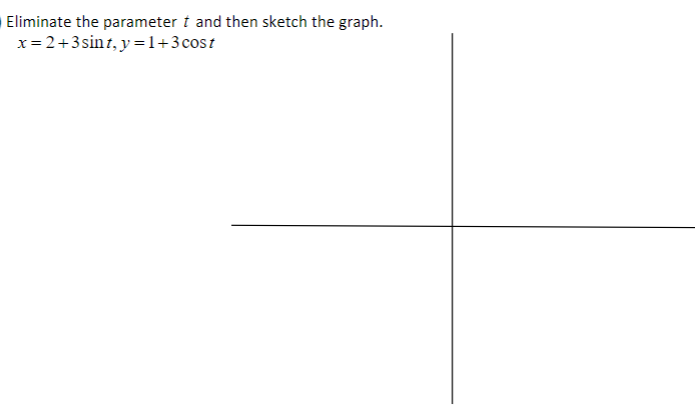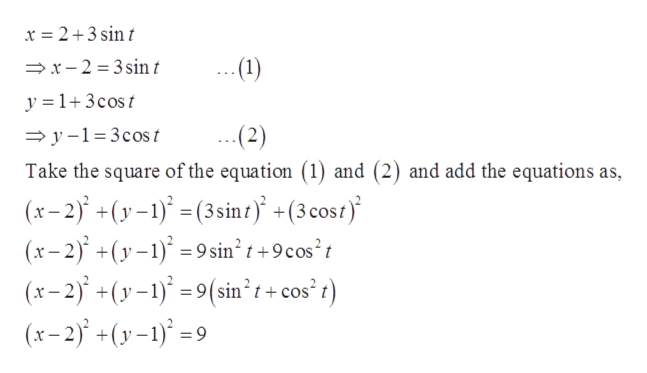# Eliminate the parameter t and then sketch the graph.1+3 costx 2+3sinr, y

Question
2 views

photo is attachedhelp_outlineImage TranscriptioncloseEliminate the parameter t and then sketch the graph. 1+3 cost x 2+3sinr, y fullscreen
check_circle

Step 1

To sketch the graph of the given parametric equation.

Step 2

Given:

Step 3

Remove the parame...help_outlineImage Transcriptionclosex 2+3 sin x-2 3sin t --(1) y =1+3cost (2) y-1 3cost Take the square of the equation (1) and (2) and add the equations as, (x-2) +(y-1) (3sint +(3cost (x-2)y1)9 sin2 t+9cos't (x-2)+(y-1)9(sin't+cos' t (x-2)+(y)9 fullscreen

### Want to see the full answer?

See Solution

#### Want to see this answer and more?

Solutions are written by subject experts who are available 24/7. Questions are typically answered within 1 hour.*

See Solution
*Response times may vary by subject and question.
Tagged in

### Other